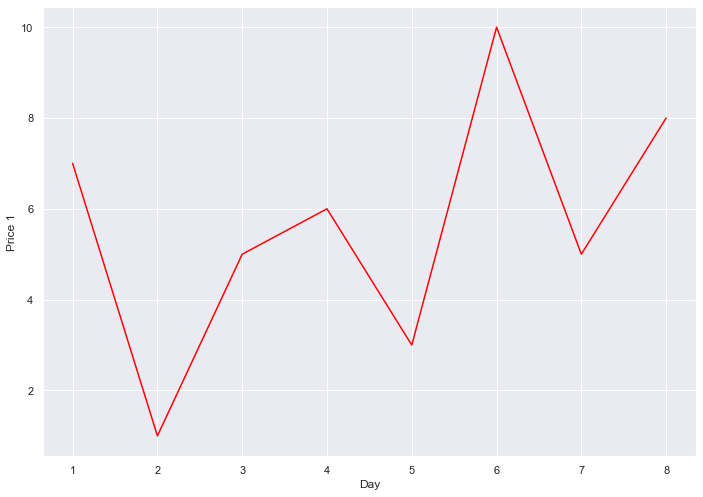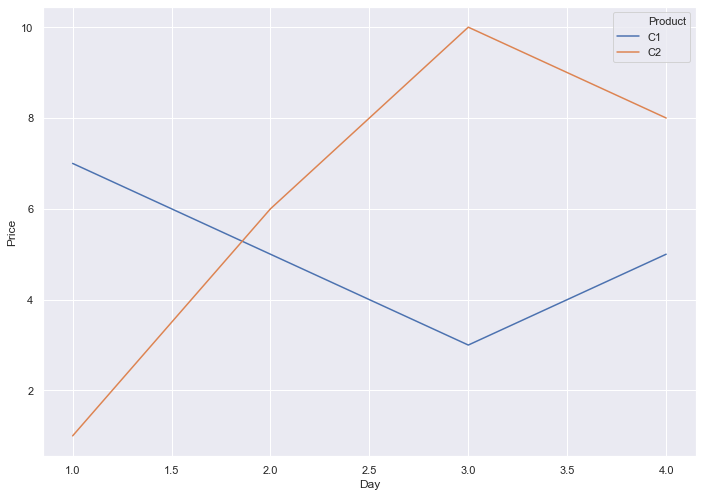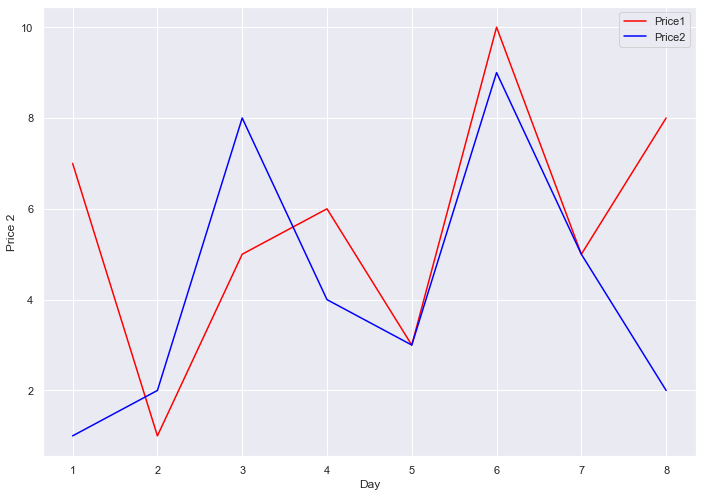# Seaborn Lineplot 线图

Seaborn 是一种高效的 Python 可视化工具，可用于创建令人惊叹的绘图。它使用并基于 matplotlib 模块。

``````import pandas as pd
import matplotlib.pyplot as plt
df = pd.DataFrame({"Price 1": [7,1,5,6,3,10,5,8],
"Day": [1,2,3,4,5,6,7,8]})
import seaborn as sns
s1 = sns.lineplot(x = 'Day', y = 'Price 1', data = df, color = 'red')
````````````import pandas as pd
import matplotlib.pyplot as plt
df = pd.DataFrame({"Price": [7,1,5,6,3,10,5,8],
"Product": ['C1','C2','C1','C2','C1','C2','C1','C2'],
"Day": [1,1,2,2,3,3,4,4]})
import seaborn as sns
s = sns.lineplot(x ="Day", y = 'Price', data = df, hue = "Product")
````````````import pandas as pd
import matplotlib.pyplot as plt
df = pd.DataFrame({"Price 1": [7,1,5,6,3,10,5,8],
"Price 2" : [1,2,8,4,3,9,5,2],
"Day": [1,2,3,4,5,6,7,8]})
import seaborn as sns
s1 = sns.lineplot(x = 'Day', y = 'Price 1', data = df, color = 'red')
s2 = sns.lineplot(x = 'Day', y = 'Price 2', data = df, color = 'blue')
plt.legend(labels = ['Price1','Price2'])
``````